# AP Board 7th Class Maths Solutions Chapter 10 Construction of Triangles Unit Exercise

SCERT AP 7th Class Maths Solutions Pdf Chapter 10 Construction of Triangles Unit Exercise Questions and Answers.

## AP State Syllabus 7th Class Maths Solutions 10th Lesson Construction of Triangles Unit Exercise

Construct triangles for the following :

Question 1.
Construct ∆PQR with measurements PQ = 5.8 cm, QR = 6.5 cm and PR = 4.5 cm.
Given measurements of ∆PQR are PQ = 5.8 cm, QR = 6.5 cm and PR = 4.5 cm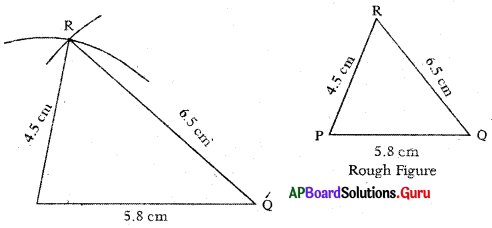Steps of Construction:

1. Draw a rough sketch of triangle arid label it with given measurements.
2. Draw a line segment with PQ = 5.8 cm.,
3. Draw ati arc with centre P and radius 4.5 cm.
4. Draw another arc with centre Q and radius 6.5 cm. to intersect the previous arc at R.
5. Join PR and QR.
Thus, required ∆PQR is constructed with the given measurements.Question 2.
Construct an isosceles triangle LMN with measurements LM = LN = 6.5 cm and MN=8cm.
Given measurements of ∆LMN are LM = LN = 6.5 cm and MN = 8 cm.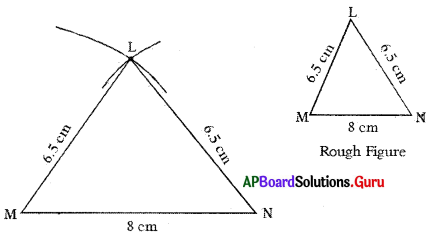Steps of Construction :

1. Draw a rough sketch of triangle and label it with given measurements.
2. Draw a line segment with MN = 8 cm.
3. Draw an arc with centre M and radius 6.5 cm.
4. Draw another arc with centre N and same radius (6.5 cm) to intersect the previous are at L
5. Join ML and NL.
Hence, required ∆LMN is constructed with the given measurements.

Question 3.
Construct ∆ABC with measurements ∠A = 60°, ∠B = 706 and AB = 7 cm.
Given measurements of ∆ABC are ∠A = 60°, ∠B = 70° and AB = 7 cm.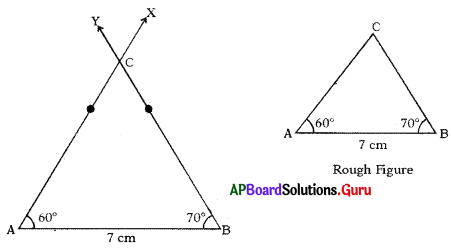Steps of Construction :

1. Draw a rough sketch of triangle and label it with given measurements.
2. Draw a line segment AB = 7 cm.
3. Draw a ray AX such that ∠BAX = 60°.
4. Draw another ray BY such that ∠ABY = 70°.
5. Name the intersecting point of AX and BY as C.
Hence, required ∆ABC is constructed with the given measurements.

Question 4.
Construct a right angled triangle XYZ in which ∠Y = 90°, XY = 5 cm and YZ = 7 cm.
Given measurements of ∆XYZ are ∠Y = 90°, XY = 5 cm and YZ = 7 Cm.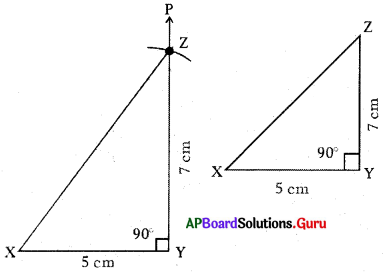Steps of Construction:

1. Draw a rough sketch of triangle and label it with given measurements.
2. Draw a line segment with XY = 5 cm.
3. Draw a ray YP such that ∠XYP = 90°
4. Draw an arc with centre Y and radius 7 cm to intersect YP at point Z.
5. Join XZ.
Hence, required ∆XYZ is constructed with the given measurements.Question 5.
Construct an equilateral triangle DEF in which DE = EF = FD = 5 cm.
Given measurements of ∆DEF are DE = EF = FD = 5 cm.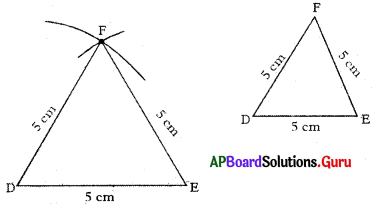Steps of Construction:

1. Draw a rough sketch of the triangle and label it with the given measurements.
2. Draw a line segment DE of length 5 cm.
3. Draw an arc with centre D and radius 5 cm.
4. Draw another arc with centre E and same radius (5 cm) to intersect the previous arc at F.
5. Join DF and EF.
Thus, required triangle ∆DEF is constructed with the given measurements.

Question 6.
Construct a triangle with non-included angle for the sides of ST and SU of lengths 6 v and 7 cm. respectively and ∠T = 80°.
Given measurements of ∆STU are ST = 6 cm, SU = 7 cm and ∠T = 80°.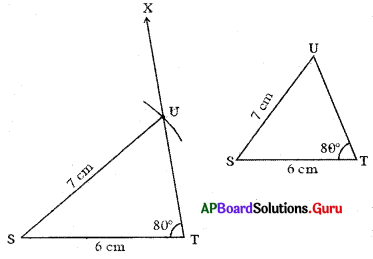Steps of Construction:

1. Draw a rough sketch of triangle and label it with given measurements.
2. Draw a line segment with ST = 6 cm.
3. Draw a ray TX such that ∠STX = 80°.
4. Draw an arc with centre S and radius 7 cm to intersect TX at point U.
5. Join SU.
Hence, required triangle ∆STU is constructed with the given measurements.Question 7.
Can you construct ∆DEF with DE = 7 cm, EF = 14 cm and FD = 5 cm. ? If not give reasons. .